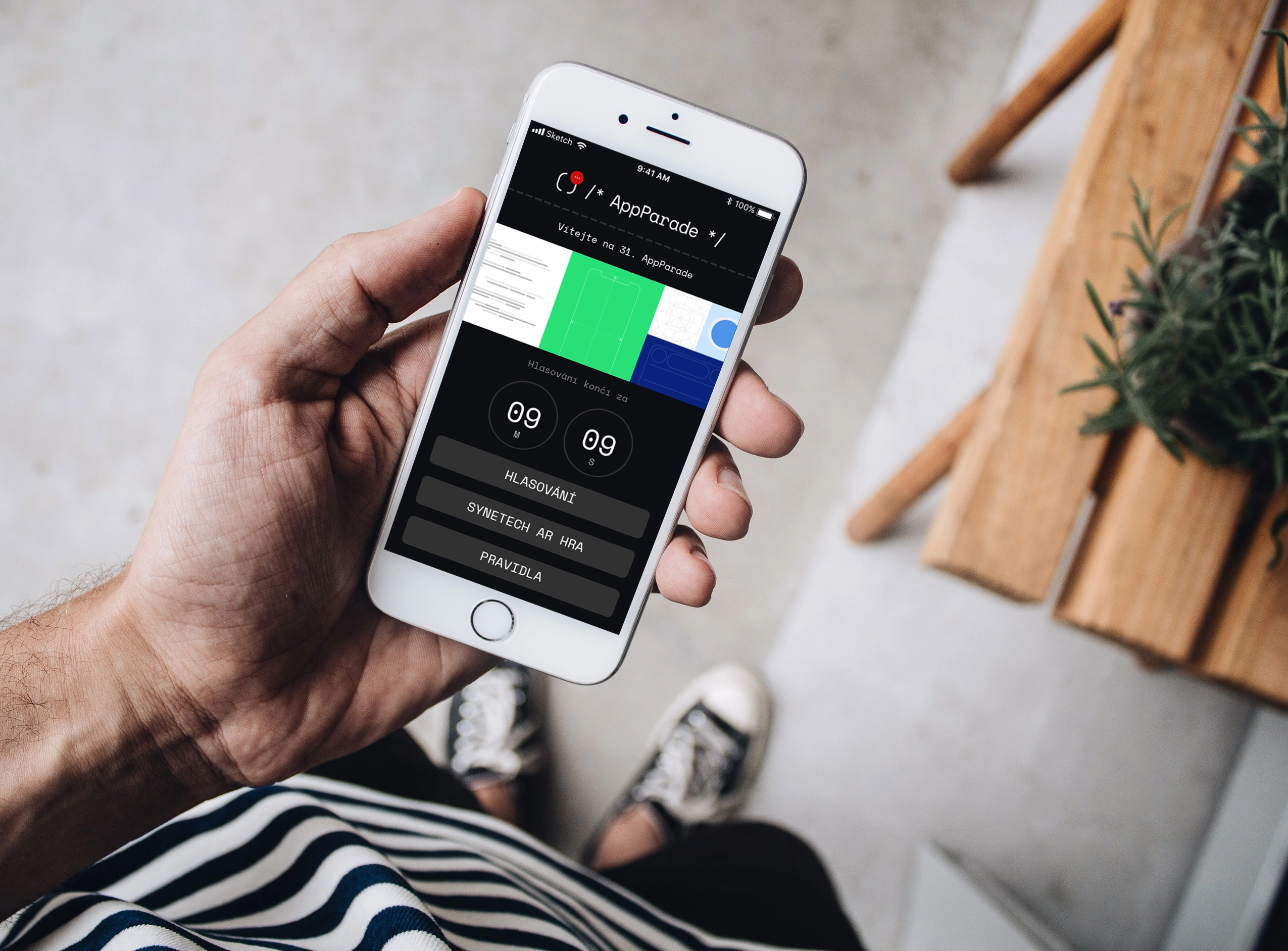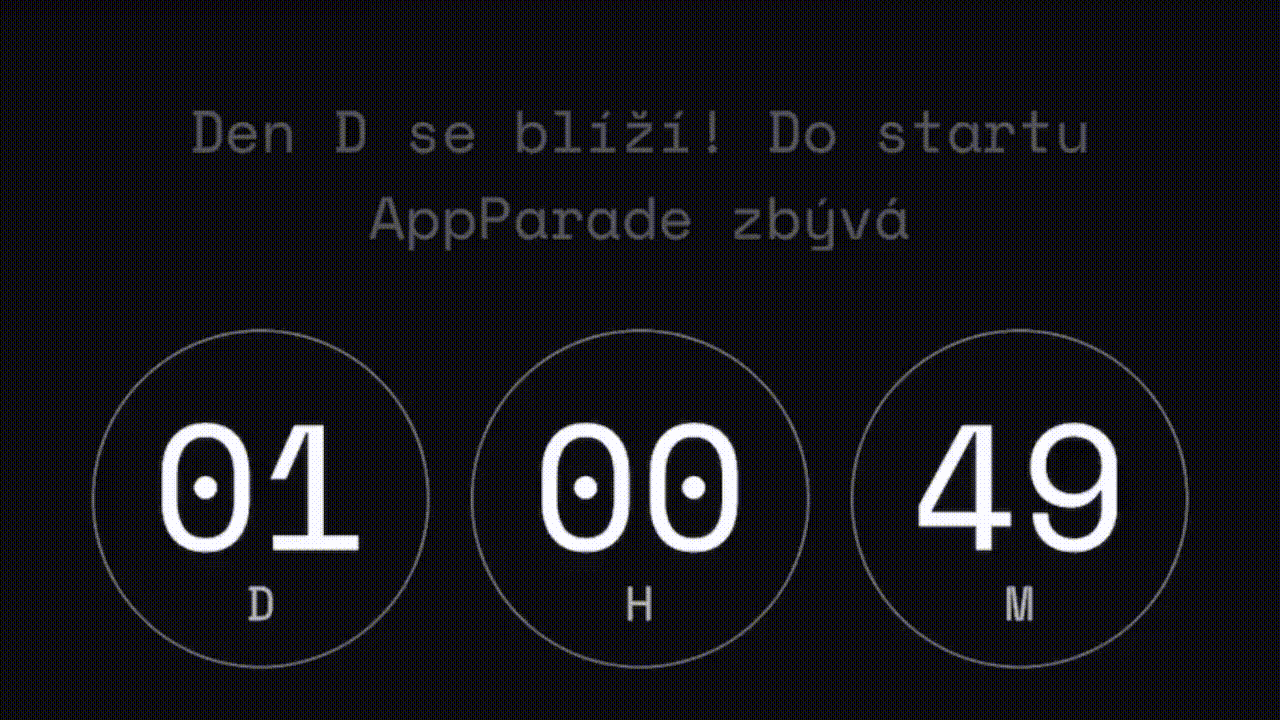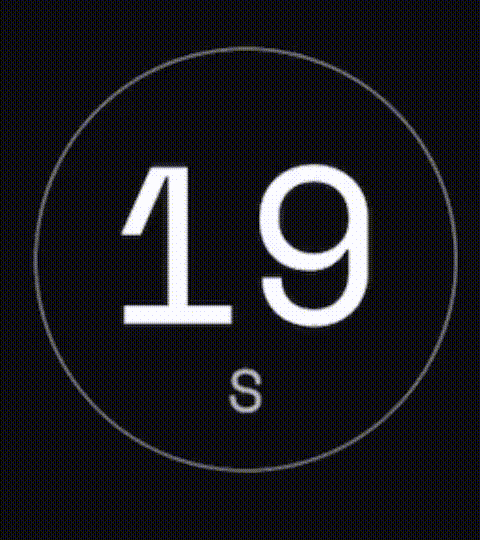## Reactive Countdown

Countdown within mobile applications is not a common feature, yet it might sometimes be very important, useful and/or user friendly when added as an application feature.Countdown within mobile applications is not a common feature, yet it might sometimes be very important, useful and/or user friendly when added as an application feature. Users can find it handy to be able to see countdown until the start of certain events, it can be used in custom timers, etc.

However, how do you actually implement something like a countdown? Subtracting seconds with `NSTimer` obviously won't do it. Also, how do you react when the target date (e.g. start of the event) changes and the application has already been released? You cannot just hardcode it to the application. This article answers all these questions, so let's get into it!

#### Foreword

Before we begin let's briefly introduce the problem we're solving.

We're hosting an event every few months called AppParade. We've developed an app for this event and before the event, it's supposed to show a countdown.

The challenge here was that the date can change any time and we want the timer to be reactive.

The following code is designed for Rx if you don't know what Rx is and how it works head over to [ReactiveX] (http://reactivex.io/intro.html) or Combine from Apple

#### The Code

Let's start by showing you how the timer looks like.The countdown depends on one input and that's the date. This is provided by a database (Firebase Firestore) and can be dynamically updated. Whenever this happens the countdown has to change seamlessly.

The goal that we are trying to achieve is to read the source only once and then just listen to updates. While doing this we want to update the UI regularly so the user has a great experience.

To achieve this the UI has to be updated more than once each second so we don't skip a second as you can see on the picture.Let's start by defining the model that represents the countdown with a number of days, hours, minutes and seconds.

``````/// Representation of a timer that can be used to display the countdown
struct Timer {
let seconds: Int
let minutes: Int
let hours: Int
let days: Int
}

extension Timer {
/// Create a timer from time interval
///
/// - Parameter interval: time interval in seconds
/// - Returns: new Timer object
static func from(interval: TimeInterval) -> Timer {
let seconds = Int(interval.truncatingRemainder(dividingBy: 60))
let minutes = Int((interval / 60).truncatingRemainder(dividingBy: 60))
let hours = Int((interval / 3600).truncatingRemainder(dividingBy: 24))
let days = Int((interval / (24 * 3600)))
return Timer(seconds: seconds, minutes: minutes, hours: hours, days: days)
}

/// Print time for debuging
func printTime() {
print("\(self.days) days, \(self.hours) hours, \(self.minutes) minutes, \(self.seconds) seconds")
}
}
``````

Next let's define a in-memory storage that provides the date to which we are counting down.

``````/// End date as it would be provided by a remote database
let endDate = BehaviorRelay < Date > (value: Date.distantFuture);
/// The observable provided by the "database"
let endDateObsevable = endDate.asObservable();
``````

and a sampling rate at which we want to update the UI (or in this example prints the result).

``````/// End date as it would be provided by a remote database
let endDate = BehaviorRelay < Date > (value: Date.distantFuture);
/// The observable provided by the "database"
let endDateObsevable = endDate.asObservable();
``````

Finally, let's do some Rx magic. We want to take the date to count to (endDate) and somehow merge it with the current time and do all this at a provided sampling rate. This can be achieved in multiple ways so let's look at two of them.

1. Use flatMap to repeat the endDate at a provided sampling rate and then use the build-in function timeIntervalSinceNow that provides the number of seconds to the specified date since now.
``````/// Rx Magic happening here
endDateObsevable
.flatMapLatest{ (endDate) in
return sampling.map { _ in
return endDate
}
}
.map { (endDate) in
return endDate.timeIntervalSinceNow
}
.map { (interval) -> Timer in
return Timer.from(interval: interval)
}
.subscribe(onNext: { (timer) in
timer.printTime()
})
``````

The output is:

To see only the latest value we have to use flatMapLatest which works the same as a flatMap however it only maps the values from the last observable. Without this all the timers would mixup when the endDate changes. Like you can see in the next video.

1. The second way how to achieve this is to use the combineLatest operator that lets you combine the latest values of two observables. The first one would be the endDate and the second one is the sampling interrupt. Then the rest is the same as in 1.
``````Observable.combineLatest(endDateObsevable, sampling)
{  (endDate, _) in
return endDate.timeIntervalSinceNow
}
.map { (interval) -> Timer in
return Timer.from(interval: interval)
}
.subscribe(onNext: { (timer) in
timer.printTime()
})
``````

If you want to save some computing power of the end device you can use the distinctUntilChanged operator that will skip values until they change and therefore the UI won't have to refresh according to the sampling rate but only if there is a change to display to the user.

Don't break the Rx chain\ If you want to save some computing power of the end device you can use the distinctUntilChanged operator that will skip values until they change and therefore the UI won't have to refresh according to the sampling rate but only if there is a change to display to the user. This solution has a few drawbacks. Firstly, you will have to take care of updating the view which is unnecessary since you have an Rx chain. Secondly, whenever the view is destructed you are still listening for the updates and therefore depleting the system resources. Finally, it can lead to an error when not syncing the view properly.

And that's the reactive countdown done. All you need to do now is to set up the remote storage from where you get the date of your event and you are set to go! You can find sample projects with implementations using the RxSwift and also Swift's new Combine in the resources below this article. Have a look and try it out your self!